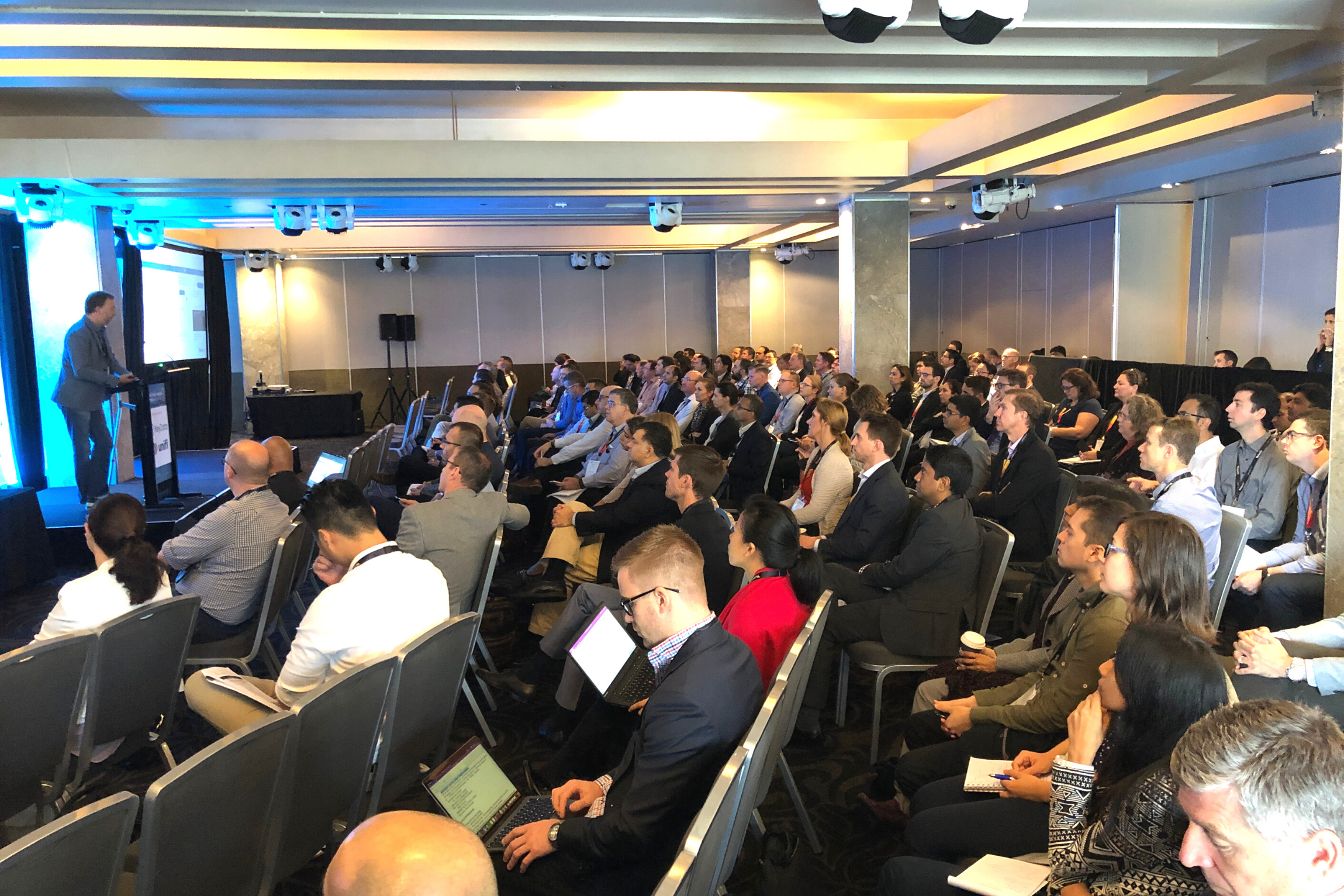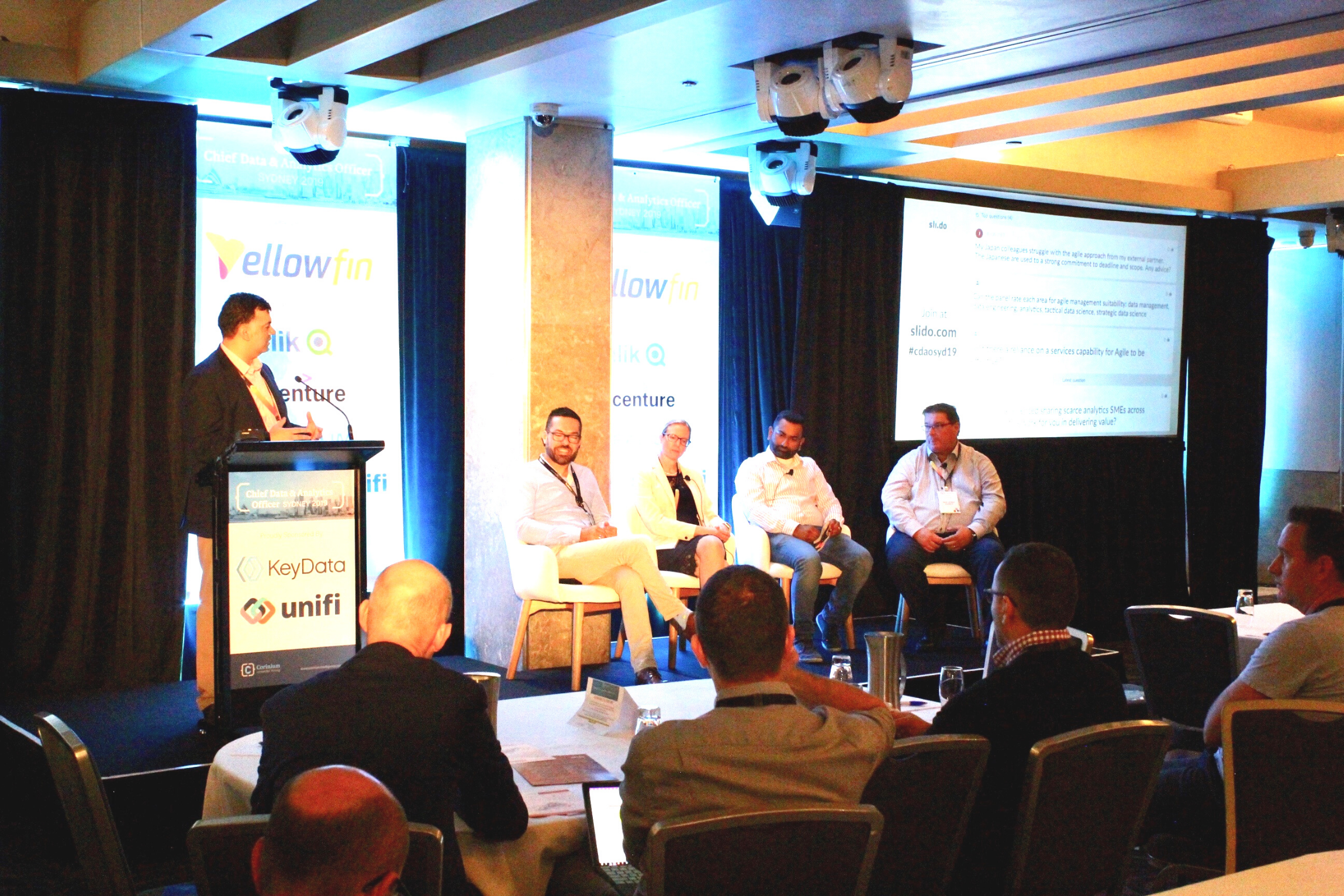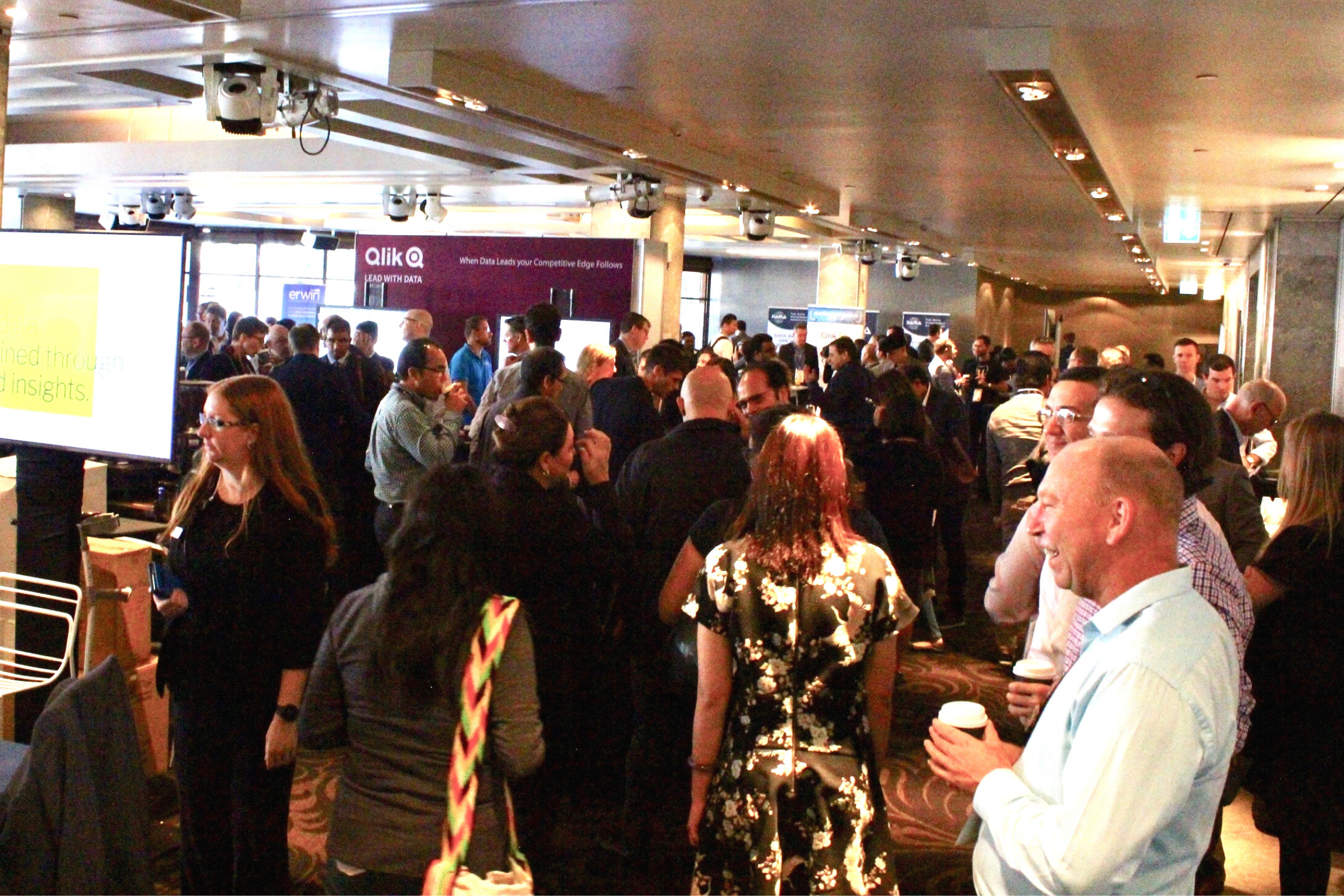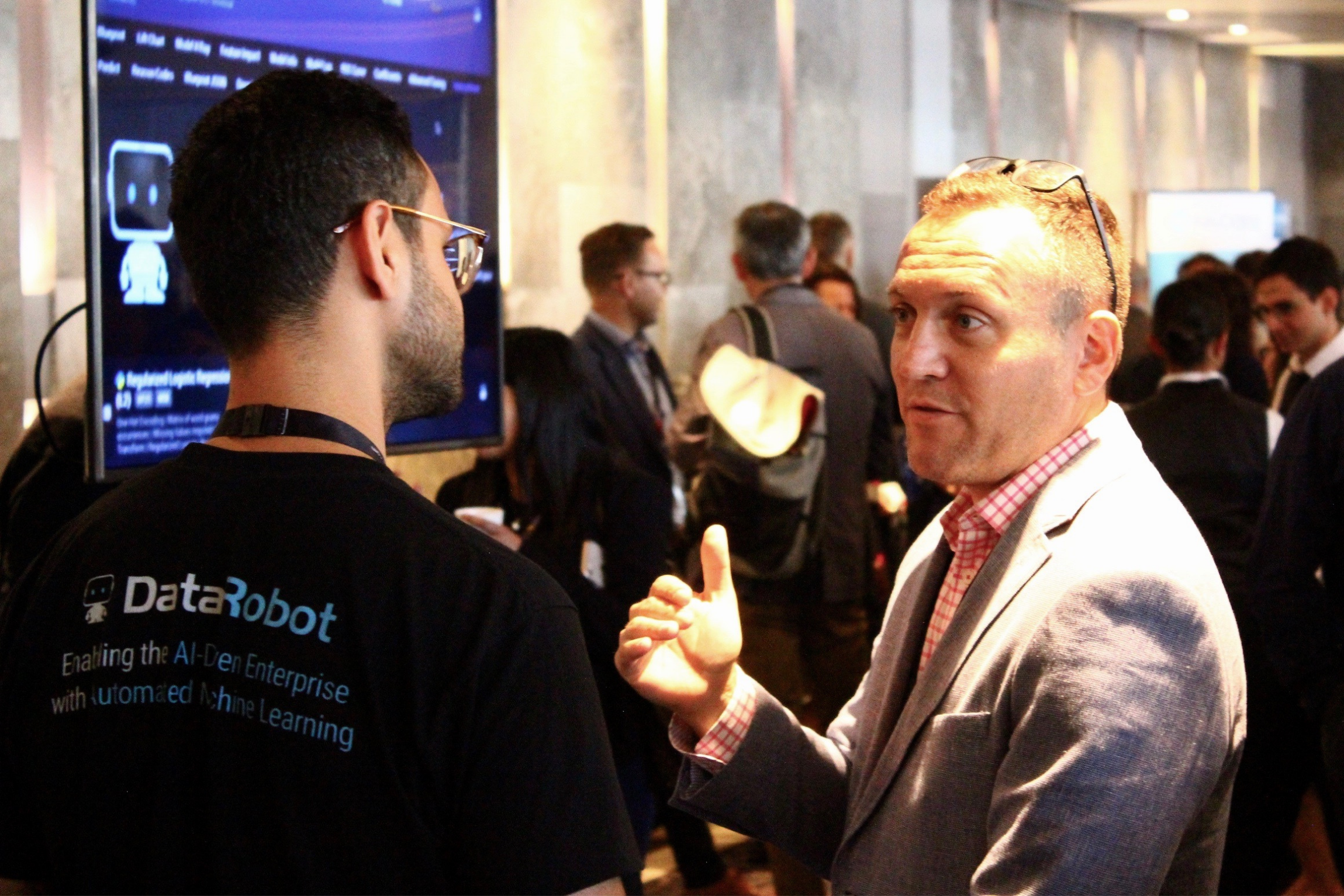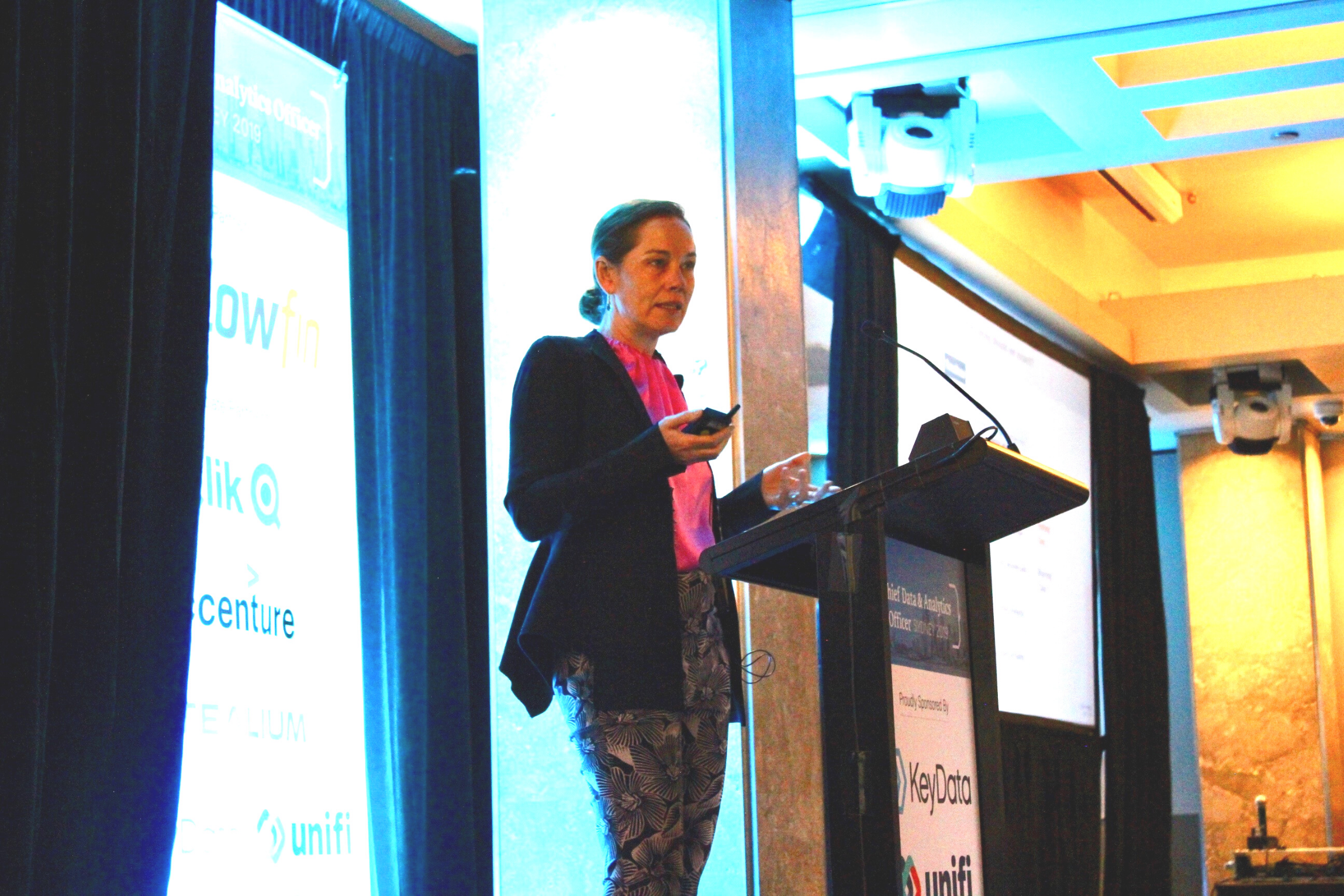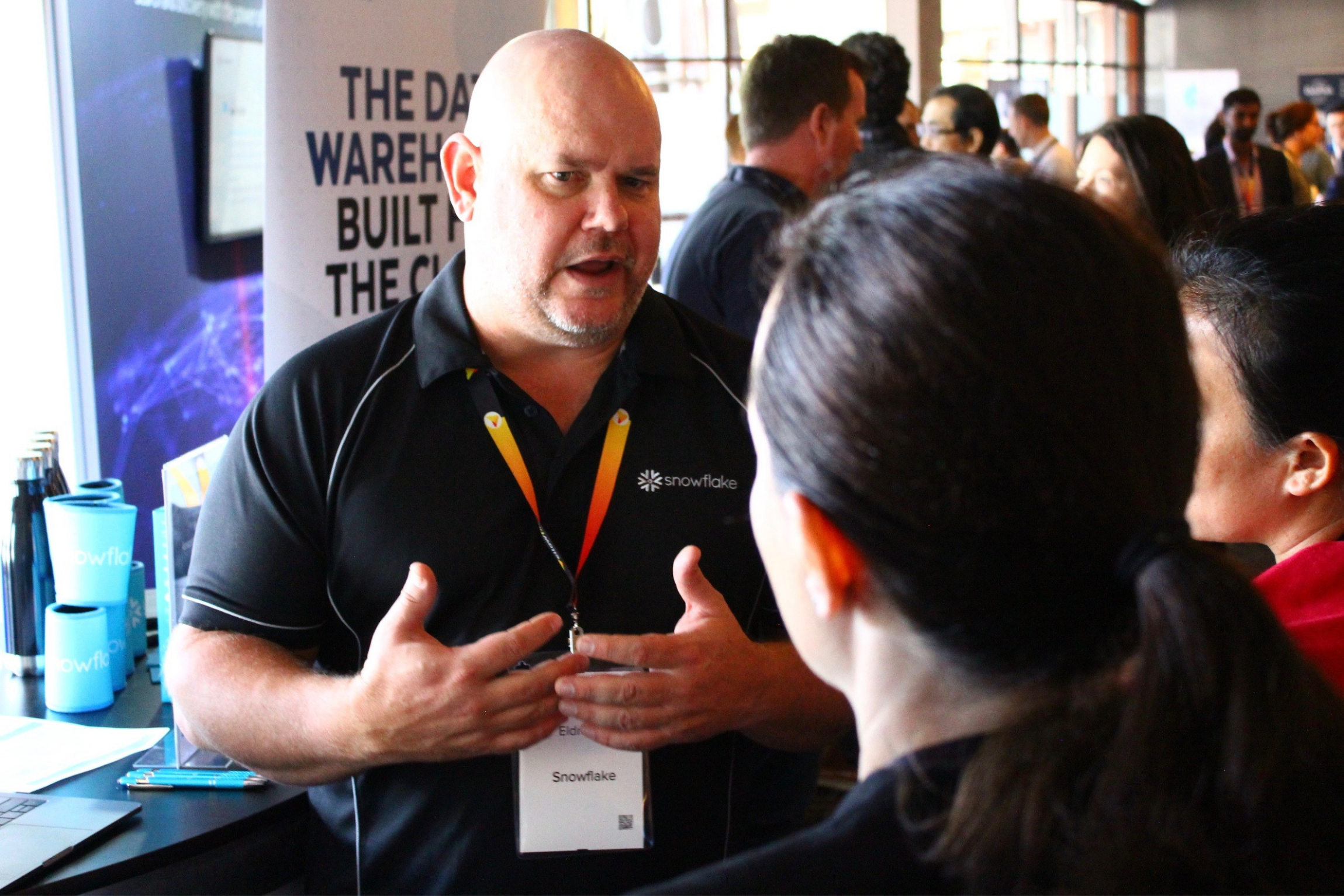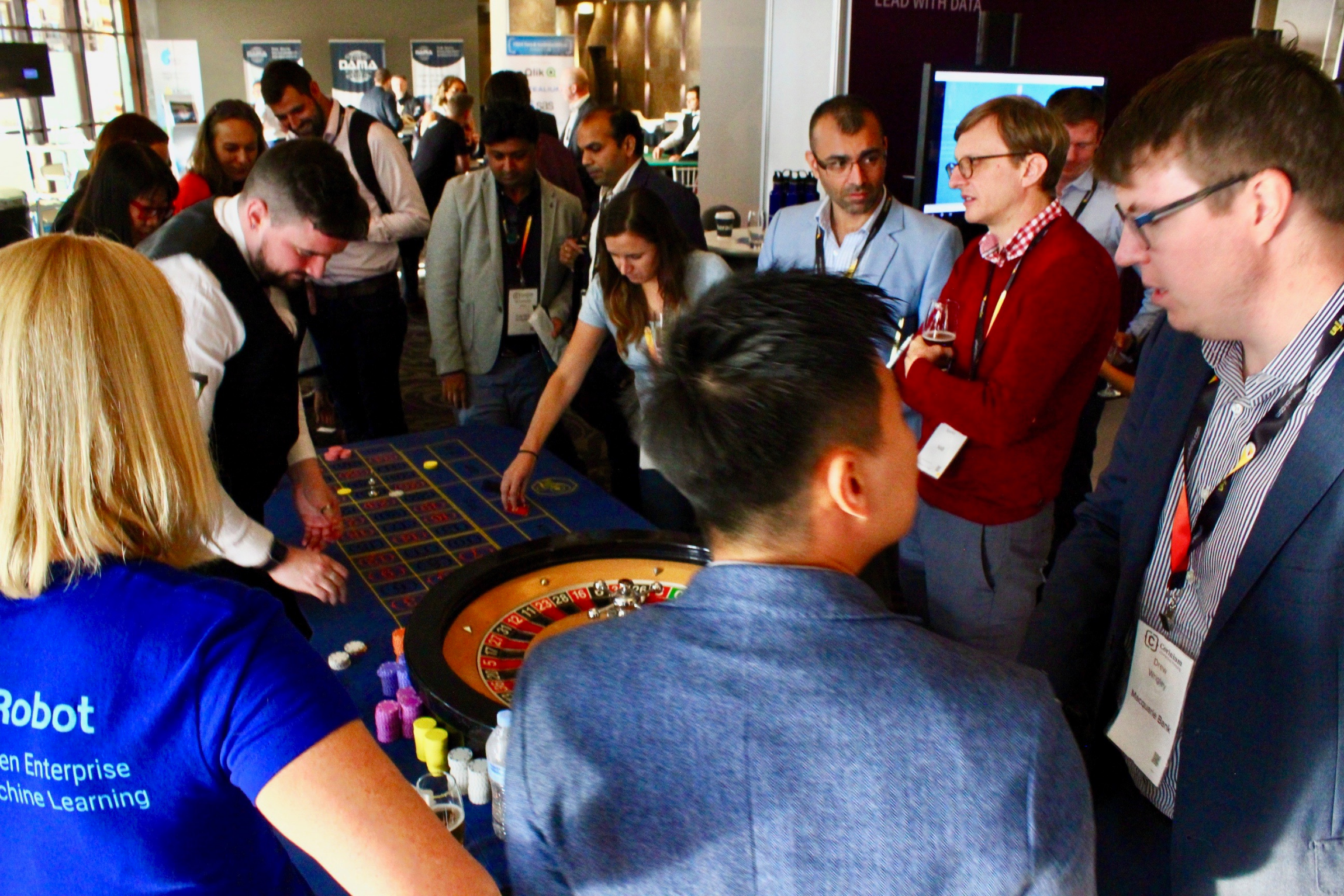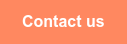<img height="1" width="1" style="display:none" alt="" src="https://www.facebook.com/tr?id=367542720414923&amp;ev=PageView&amp;noscript=1">## Be inspired, collaborate, disrupt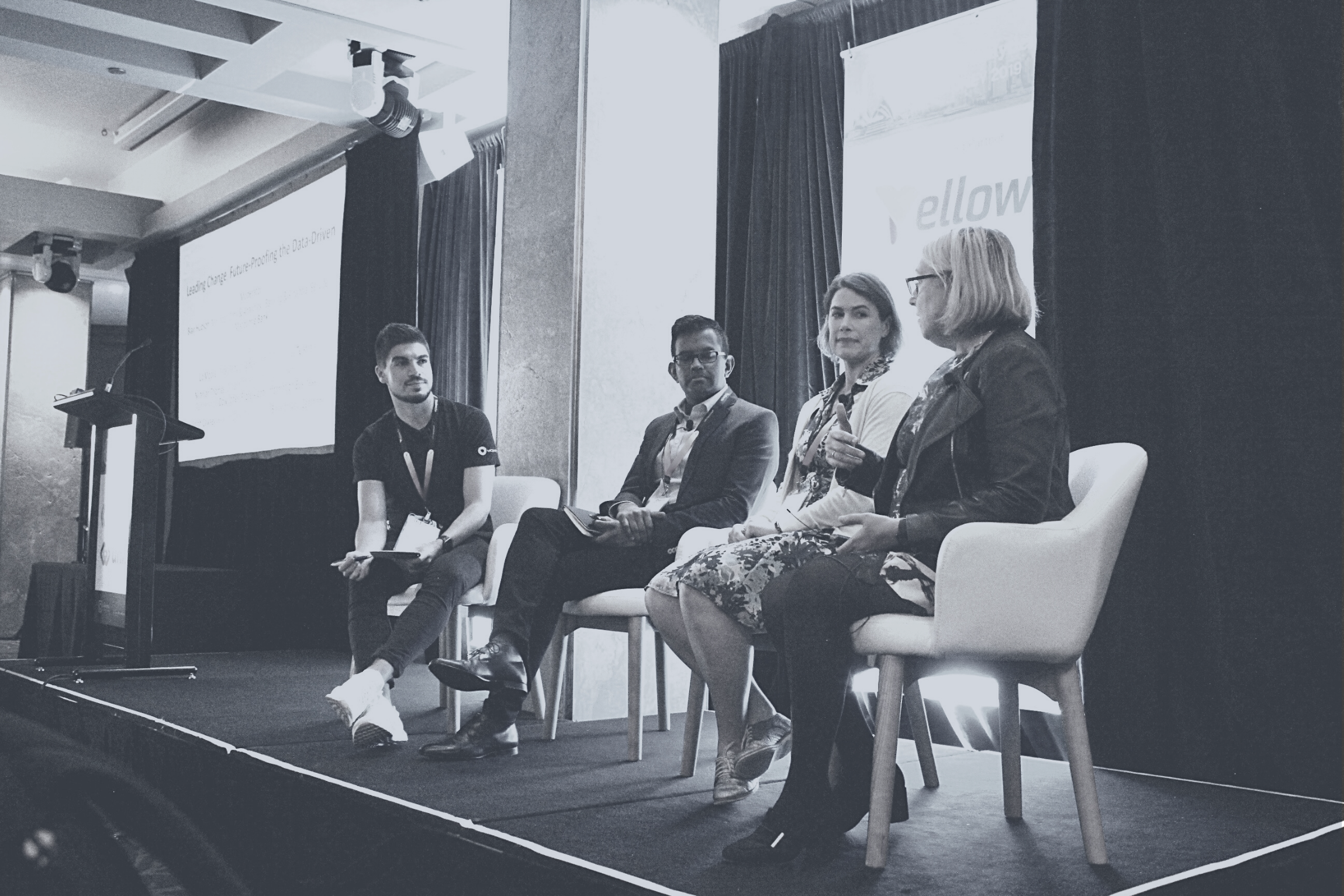Australia's senior executives responsible for championing the data agenda will gather at CDAO Sydney 2022 to share insights on transformative leadershipdata driven culture, developing high performing analytics teamsdata literacy and effective governance of data and AI.

Focusing on the biggest challenges faced today to effectively harness data as a strategic asset, drive change and shape organisations to become analytically mature, business-driven enterprises that outperform their competitors.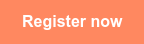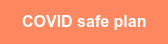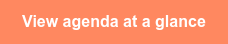12

## Hear from our expert speakers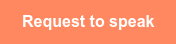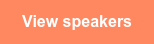## 2022 Key themes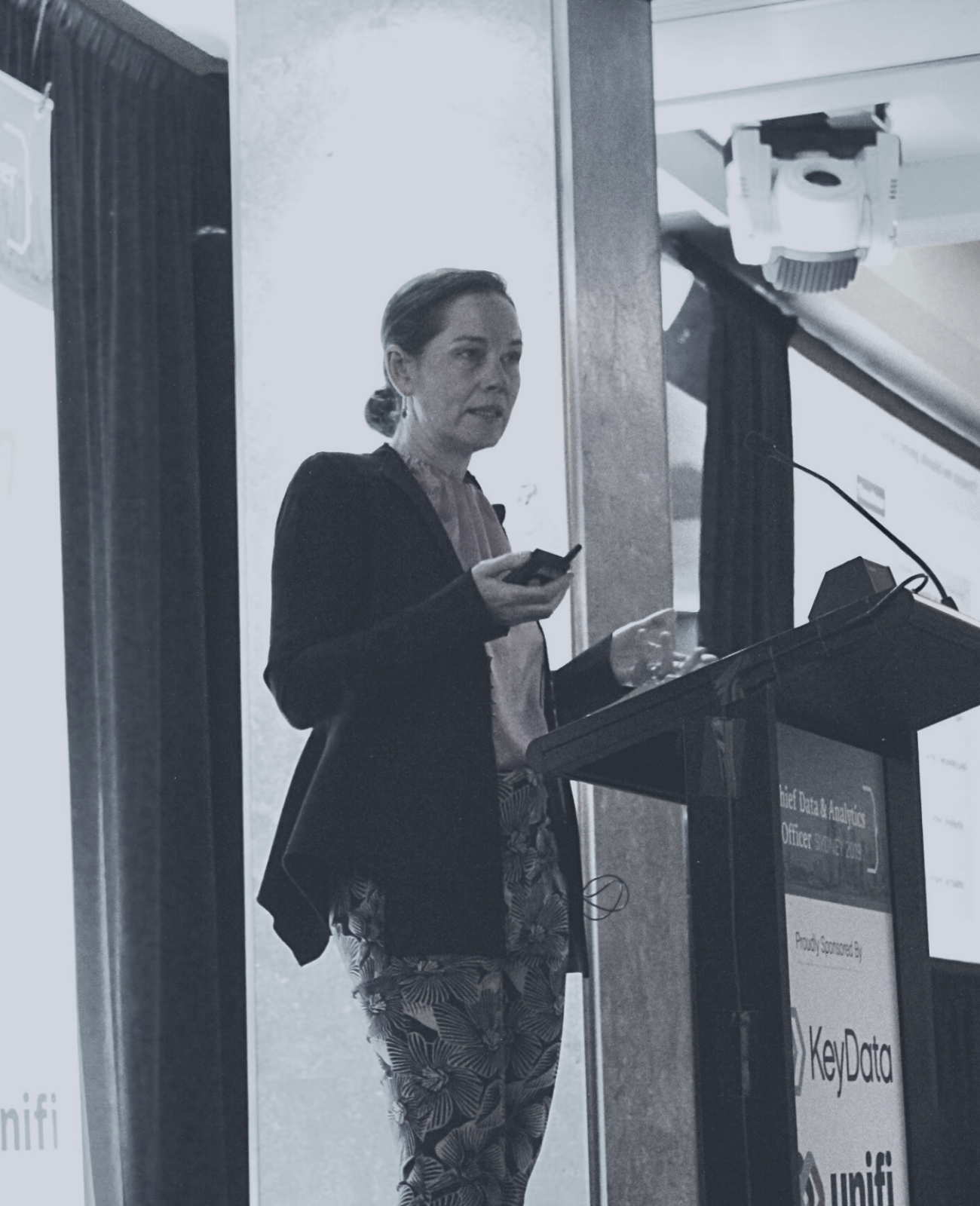Sustain change and scale for success
Ensure your strategy, foundational capabilities and activities are all geared towards embedding analytics into the fabric of the organisation.

Data-informed
Upskill, adapt and evolve. Learn how organisations are enabling change through entire cross-functional, data literate, agile teams.

Awaken data investment and opportunity, and treat data as a strategic asset
Turn your analytics narrative into a success story. With data analytics at a crossroads it’s time to separate the grain from the chaff and ensure value.

Thrive through change: Achieve data and analytics success in a post-COVID world
During the COVID-19 pandemic, data was the lifeblood of every country’s response. This event will look at how data was used, and how it will be deployed in the post-COVID world.

Effective risk management for data
Understand specific instruments and tools that can be deployed to operationalise data governance and ensure security, privacy and the ethical use of data and AI.## FEATURED REPORT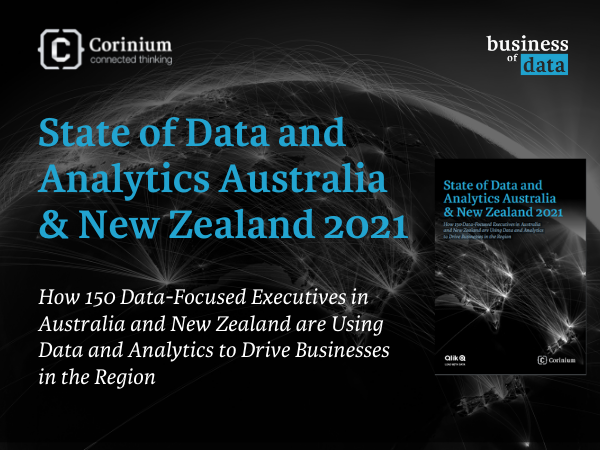What is the State of Data and Analytics in Australia and New Zealand? We Ask 150 Executives

Data and analytics is driving the digital transformation of organisations all over the world, and we want to understand how important these practices are in Australian and New Zealand.

This survey of 150 data and analytics executives explores the extent to which Australian and New Zealand organisations uses data and analytics.

Our report looks at the impact of the coronavirus pandemic on data and analytics adoption, the maturity of the data and analytics in the region, and plans for data leaders into the future.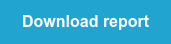## Experience CDAO Sydney's onsite events

Connect and learn even faster at our onsite events. Every year we curate new experiences at the event to enhance relationships and spark ideas outside of the session room.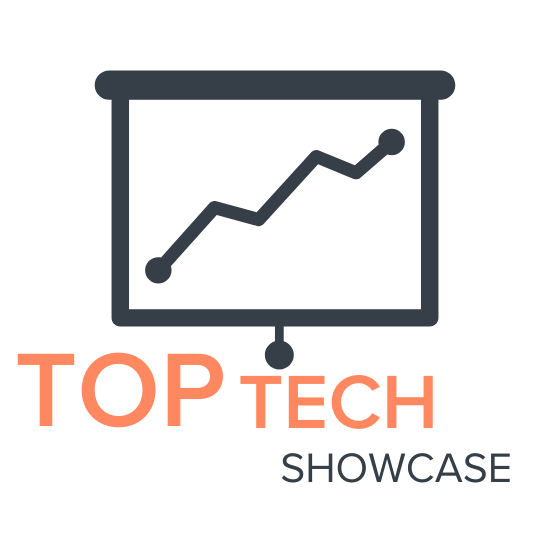Be inspired by those delivering change and serving the community through exciting new services.Two organisations will take you through their project for 15 minutes, no waffle all substance.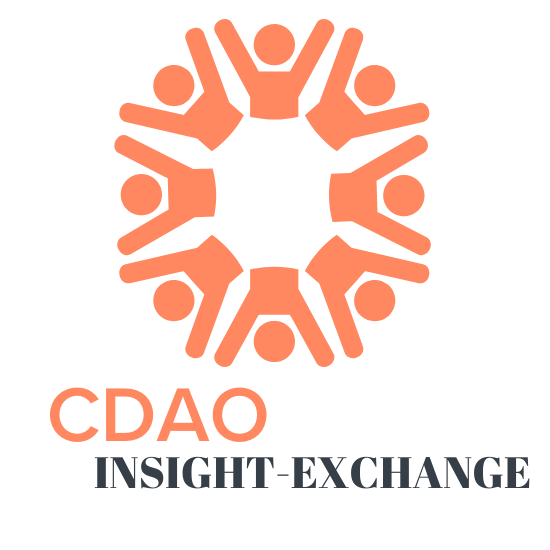Meet up with peers to discuss topics that most interest you!Expand your C-level network at our exclusive, must-attend dinner.Bring discussions to life and have common problems solved in our no holds barred discussion groups.Relax, continue the conversation during an informal and fun cheers with peers.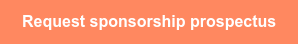## Our 2022 supporting partners

true true true true true true true true true true true true true true true true true true true true true true true true true true true true true true true true true true true true true true true true true true true true true true true true true true true true true true true true true true true true true true true true true true true true true true true true true true true true true true true true true true true true true true true true true true true true true true true true true true true true true true true true true true true true true true true true true true true true true true true true true true true true true true true true true true true true true true true true true true true true true true true true true true true true true true true true true true true true true true true true true true true true true true true true true true true true true true true true true true true true true true true true true true true true true true true true true true true true true true true true true true true true true true true true true true true true true true true true true true true true true true true true true true true true true true true true true true true true true true true true true true true true true true true true true true true true true true true true true true true true true true true true true true true true true true true true true true true true true true true true true true true true true true true true true true true true true true true true true true true true true true true true true true true true true true true true true true true true true true true true true true true true true true true true true true true true true true true true true true true true true true true true true true true true true true true true true true true true true true true true true true true true true true true true true true true true true true true true true true true true true true true true true true true true true true true true true true true true true true true true true true true true true true true true true true true true true true true true true true true true true true true true true true true true true true true true true true true true true true true true true true true true true true true true true true true true true true true true true true true

## Register today!

Australia's senior executives responsible for championing the data agenda will gather at CDAO Sydney 2022 to share insights on transformative leadershipdata driven culture, developing high performing analytics teamsdata literacy and effective governance of data and AI.

Focusing on the biggest challenges faced today to effectively harness data as a strategic asset, drive change and shape organisations to become analytically mature, business-driven enterprises that outperform their competitors.

## What people are saying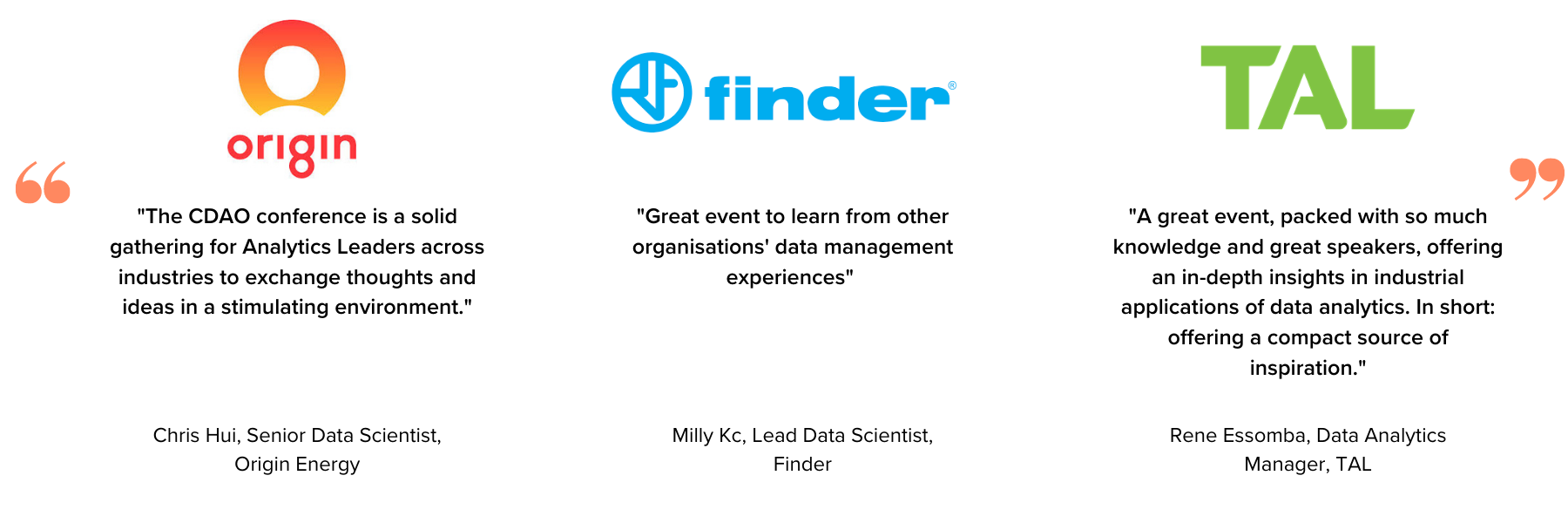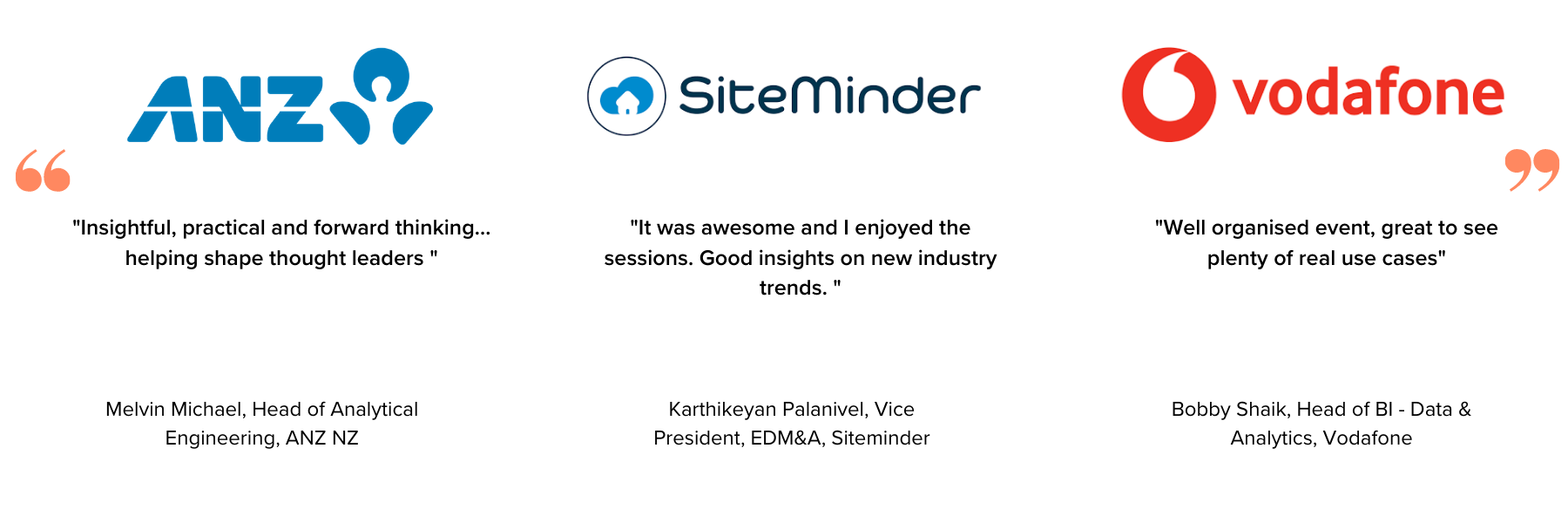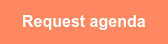## Previous events gallery